You are browsing international version of the website. Based on location data, the suggested version of the page for you is USA / US
Register

# Induction coil and its application in the practice of an electronic engineer

2020-11-11

Despite the widespread use of digital circuits, such as processors, programmable logic devices and SoC circuits, being a combination of the two, sometimes the electronics designer has to reach for ‘analogue’ elements, such as: resistors, capacitors or induction coils. What is interesting, while it is relatively easy to incorporate a resistor or a capacitor (with a capacity counted in picofarads) into an integrated circuit structure, it is very hard to do so with an induction coil. That is why the application notes of many elements still mention an induction coil as an external component added to the set. This article offers some basic information about induction coils and a description of elements of their structure that affect their parameters.

## Structure of an induction coil

An induction coil is not complicated. It consists of a core and insulated wires wound around it. The core can be either an air core or one made from magnetic materials. It is important that the wires wound around the core are insulated, which is why insulated wire is used to make coils, or they are wound using an uninsulated wire (for example, the so-called silver steel), but with an air gap ensuring the required separation between the individual turns of the wire. If uninsulated wire is wound turn by turn, a short-circuit will occur and, although some inductance will be present, it will definitely be different from the desired one.

In practice, damage to the induction coil often occurs, i.e. a short-circuit between the wire turns as a result of insulation breakdown, due to exceeding the maximum permissible temperature or voltage. A coil damaged in this way needs to be rewound or replaced with a new one. Mains transformers also get damaged in this way. Further use of such a damaged transformer can result in its overheating, a short-circuit in the mains or even ignition of the transformer or a device powered by it.

## What is an induction coil?

An induction coil is an element that stores energy as a magnetic field in the core, so it changes the electric current energy into the magnetic field energy, or the other way round. Change of the current flowing through the windings leads to the generation of an electromotive force in the direction that counteracts this change. Similarly, the changeable magnetic field penetrating the core causes the induction of voltage. This can be shown using the following formula: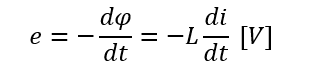In this formula:

• e - stands for the electromotive force (voltage in volts) generated by the coil,
• dϕ/dt - stands for the magnetic flux change in time,
• di/dt - stands for the current change in time,
• L - stands for a coil parameter called inductance; its unit is henry.

It is easy to spot the feature that was mentioned earlier – the electromotive force e has an opposite direction than the voltage causing the current flow. It counteracts rapid changes of current flowing through the coil and enables the coil to perform one of its basic functions – to be used as a so-called impeder.

## Induction coil – basic parameters

Basic parameters of a coil are its inductance and resonant frequency. Inductance is, in other words, the ability of the coil to store energy in the form of a magnetic field caused by the flow of the current. Inductance is measured in henries and is defined as a ratio of temporary voltage to current change in time.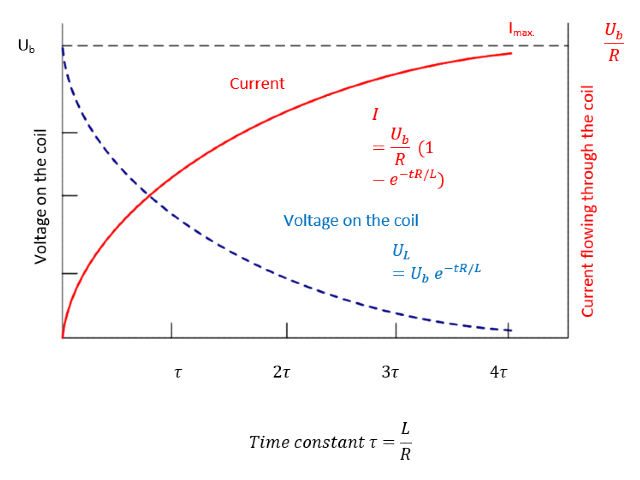Diagrams showing the current and voltage drop on the induction coil terminal. The drop is greatest at the moment of activating the power supply and decreases with time. The drop counteracts the increase of the current, hence, the amperage is the lowest at the moment of activating the power supply and increases with time. It is often said that the voltage leads the current on the coil

The figure above shows what happens with the voltage on the coil and with the current flowing through it after power is supplied to its terminals. The solid red line illustrates the flow of the current. As we can observe, the current increases once the power is supplied, until its peak value as defined by the Ohm’s Law, i.e. the ratio of the voltage on the terminals to coil resistance, is reached. The dashed blue line illustrates the drop of voltage on the coil. As we can observe, this drop is greatest at the moment the power is supplied and it is lowest after the current reaches its peak value. It is connected with the fact mentioned earlier, that the induction voltage has the opposite direction than the voltage applied to terminals.

The resonant frequency of a coil is discussed while describing the parameters of an imperfect coil, because it is connected with parasitic capacitance.

### The core material and relative magnetic permeability

A very important element of an induction coil is its core. The core is characterized by the type of material used and the relative magnetic permeability connected with it. It is called ‘relative’, because it is determined in relation to the permeability of vacuum. It is a dimensionless number defined as a ratio of the magnetic permeability (absolute μ) of a given medium to the permeability of vacuum μ0.

According to the definition, magnetic permeability is an ability of a given material or a medium to change the magnetic induction along with a change of magnetic field strength. In other words, permeability is a feature of a material or a medium describing its ability to concentrate the lines of the magnetic field.

Magnetic permeability is, in accordance with the data published in 2002 by the Committee on Data for Science and Technology (CODATA), a scalar which is denoted by μ0 symbol and whose value in the International System of Units (SI) is μ0 = 4·Π·10-7= ca. 12.566370614·10-7 [H/m = V·s/A·m].

The inductance of the coil is expressed using the following formula: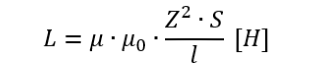The symbols used in the formula stand for:

• L - inductance in henries,
• μ0 - magnetic permeability of vacuum,
• μ - relative permeability of the core material,
• Z - number of wire turns in the coil,
• S - cross-sectional area of the coil,
• l - length of the coil.

The relative permeability of unpolluted air does not differ much from the permeability of vacuum, so, to simplify, in the engineering practice it is assumed that μ = 1 and the air-coil inductance formula is: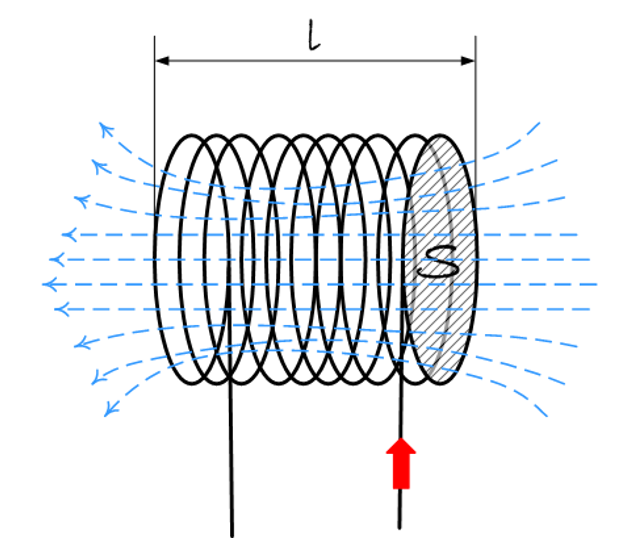Blue lines show the lines of magnetic field force directed in accordance with the Lentz Rule (the so-called right-hand rule).

In terms of magnetic features, the materials are divided into paramagnetic materials (materials which turn into magnets after they are placed in the magnetic field), ferromagnetic materials (which become magnetized in the presence of a magnetic field) and diamagnetic materials (weakening the magnetic field). The type of core material strongly affects the coil's parameters. In perfect vacuum, there are no particles which could influence the correlation between the inductance and the strength of the magnetic field. Having said that, in every material medium the inductance formula will be modified depending on the permeability of this particular medium. In the case of vacuum, the permeability value equals 1. For paramagnetic materials, the relative permeability is slightly higher than 1, for diamagnetic materials it is slightly less than 1 – the differences in both cases are so small that in technical applications they are neglected and the value is assumed to be equal to 1.

Let us summarize this paragraph by listing the parameters of the coil which have the most significant influence on its inductance:

• The coil’s inductance increases along with:

• the number of wire turns,
• the relative permeability of the core material,
• the coil’s surface area,
• decrease in the coil’s length.
• The coil’s inductance decreases, when:

• the number of wire turns decreases,
• the relative permeability of the core material decreases,
• the surface area decreases,
• the coil’s length increases.

Why are the cores used? Firstly, thanks to that it is possible to store more energy with fewer turns than in the case of an air core equivalent. Secondly, it is due to the mechanical structure of the coil – the core is a support for wire turns and enables proper mounting in a target device. The third important reason is the concentration and conduction of the magnetic field. In some applications a possibility to regulate the coil’s inductance by changing the core’s position in relation to the wire turns, for example by inserting or ejecting it, will also be important.

## An imperfect coil

Up to this point, we have been discussing the parameters of a perfect coil. Meanwhile, under realistic conditions, the winding wire will have some resistance and capacity, which will influence the coil’s actual parameters which we have not considered yet.

The figure shows an equivalent direct current scheme of a real coil. A resistor, representing the winding wire’s resistance, has been connected in series to the coil turns. With the current flowing through the coil, it will cause not only the voltage drop, but also power loss in the form of heat, which may cause the coil to overheat and change the core parameters. As a consequence, electrical efficiency of the whole device also decreases.Alternative DC schematic diagram of a real coil

In the case of alternating current analysis, the parasitic capacitance generated by the uninsulated layers of the conductor should also be taken into account, and that is why the equivalent diagram, apart from the resistor, includes also a capacitor connected in parallel to the coil’s terminals. An RLC circuit is created this way, and the coil itself is inductive before reaching the resonant frequency, and becomes capacitive after reaching it. That is why the impedance of the coil increases with the resonant frequency to reach its maximum value in resonance and decrease after exceeding the frequency.Change of a real coil from inductive to capacitive after reaching the resonant frequency. The symbols on the equivalent schematic diagram: L – inductance, EPC – parasitic capacitance, EPR – parallel resistance symbolizing the power loss, ESR – serial resistance symbolizing the winding core’s resistance)

### Three types of power losses in the induction coils

In the applications of coils, there are three dominant types of power loss considered. The first one has already been mentioned earlier, namely the loss occurring in serial resistance, i.e. that of a winding wire. This power loss should be especially taken into account when the current flowing through the coil has a high amperage. This is the most common power loss in power supplies and power circuits. It causes overheating of the coil and, as a consequence, of a whole device. This is also the most common reason for damage, as high temperature can cause harm to the insulation and a short-circuit on the coils.

The second type of power loss is that occurring in the core. It is a result of irregularities in workmanship, occurrence of eddy currents and changes in the position of magnetic domains. Such losses are dominant when the current flowing through the coil has a low amperage. They occur in circuits with a high frequency, digital signal separators and others. This may lead not so much to damage to the coil, as to loss of the signal level in sensitive circuits.

The third type of power loss is the one occurring as a result of a loss of magnetic flux which can be dispersed by mechanical mounting elements, air-gaps in the core or negligent workmanship during production of the coil itself.

Discover our offer

## Final remarks

An induction coil is a simple component and is, therefore, a little neglected. Meanwhile, when mounting an electronic circuit equipped with chokes or transducers, one should pay particular attention to the inductive components chosen, including their resonant frequencies or parameters of the core material. Different cores are used with the current frequency of tens or hundreds of hertz and different ones with a frequency of hundreds of megahertz and more. Sometimes, with high frequency signals, a ferrite bead is enough.

Induction coils can be produced in different ways. Typically, several to several hundred turns of wire are wound on the core. In some applications, turns are wound as paths on a printed board, and sometimes closed in a ferrite cup core. These days, the majority of coils, in particular chokes used in power circuits, are made for the purpose of SMT mounting. Yet, the technological race is tight, and ever new magnetic materials are being developed that are able to retain their characteristics and limit the losses, despite the temperature increase etc.

A coil designed to work at a low frequency typically has an iron core and a high number of turns, which makes it relatively heavy. That is why in many applications, in particular those vulnerable to shock and surges, the method of mounting plays a significant role. Usually, soldering the coil is not enough – its core has to be fixed properly using a clip, a holder or a screw. When choosing a coil or a transducer for a device, this aspect is worth taking into account.

## Applications of induction coils in electronics

Coils are used to:

• block the flow of the alternating current in the circuit,
• short-circuit the direct current (voltage),
• measure time on the basis of decline of the current flow,
• build an oscillatory circuit,
• build filters for particular frequencies,
• couple the amplifier stages,
• decrease or increase the voltage.

Some applications of coils are similar to the those of capacitors. As we already know, a coil behaves like a capacitor after exceeding the resonant frequency. It does not mean, however, that those elements can be used interchangeably in a circuit.

Make sure you watch a video concerning the topic of induction coils and its application in electronics: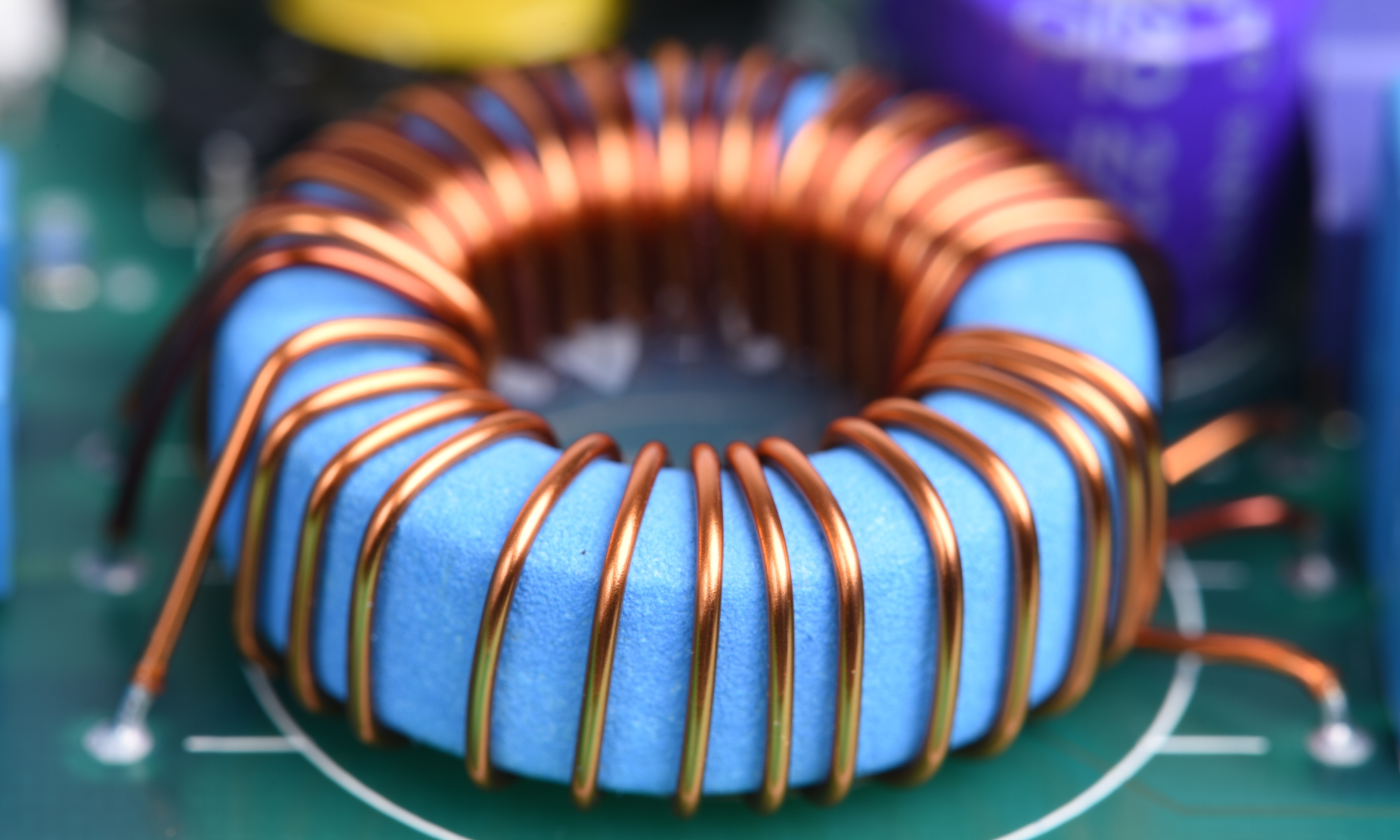ChromeFirefoxOperaInternet Explorer